# 2. Given the following three transactions T1 = r1(x); w1(y); T2 = r2(z); r2(y); w2(y); w2(x); T3 ...

2. Given the following three transactions

T1 = r1(x); w1(y);
T2 = r2(z); r2(y); w2(y); w2(x);

T3 = r3(z); w3(x); r3(y);

Consider the schedule
S = r1(x); r3(z); r2(z); w3(x); r2(y); r3(y); w2(y); w1(y); w2(x);

a. Draw the precedence graph of schedule S, and label each edge with data item(s).
b. Based on the precedence graph, determine whether S is conflict serializable and justify your answer. If it is serializable, specify all possible equivalent serial schedule(s).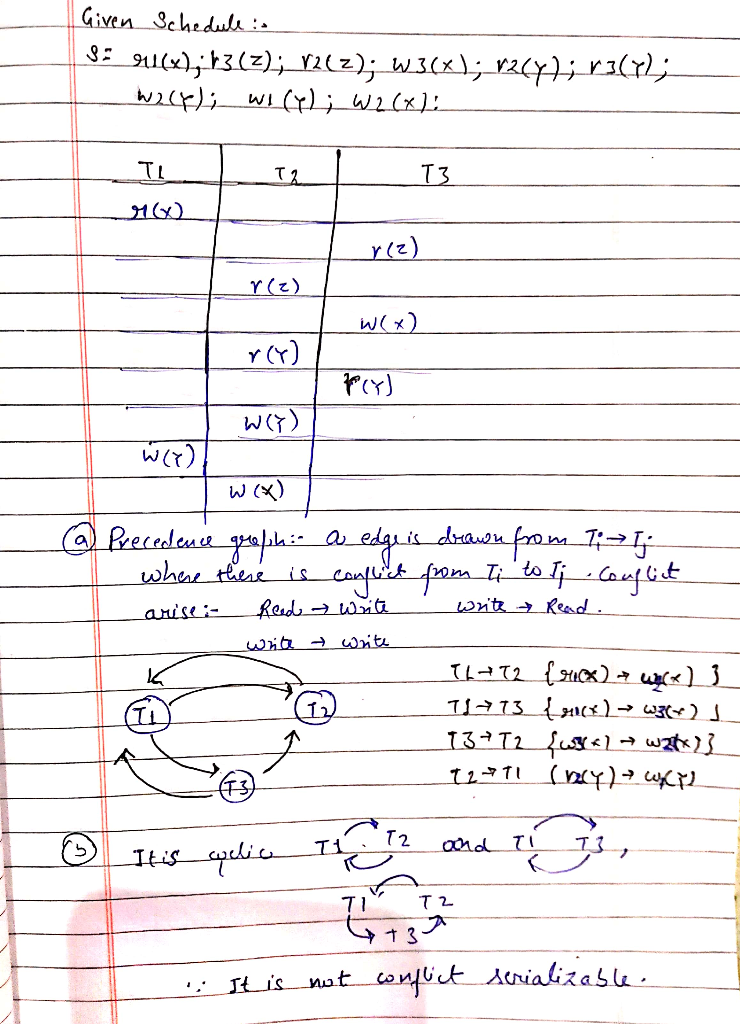##### Add Answer of: 2. Given the following three transactions T1 = r1(x); w1(y); T2 = r2(z); r2(y); w2(y); w2(x); T3 ...
Similar Homework Help Questions
• ### You are given with the following schedule, that consists of three transactions (T1, T2, T3) and...

You are given with the following schedule, that consists of three transactions (T1, T2, T3) and three database elements (A, B and C) w3(A); r1(A); w1(B); r2(B); w2(C); r3(C) a) Identify all the conflicts (as defined in Section 18.2.1 in the book). Hint: draw a bipartite graph between transactions and elements, as done in class b) Draw a precedence graph (as defined in Section 18.2.2 in the book). Is the schedule conflictserializable? Justify your answer

• ### 2. Let Wi-((a, b, c) : a-c-b), W2-((a, b, c) : ab>0), W3-((z, y,z) : r2+92+22£1} be subsets of R3 (a) Determine which of these subsets is a subspace of R3. Justify your answer. (b) For the subsets...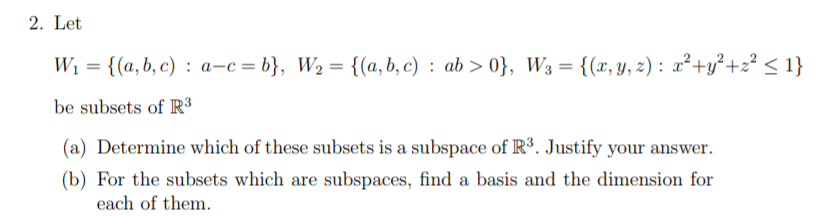2. Let Wi-((a, b, c) : a-c-b), W2-((a, b, c) : ab>0), W3-((z, y,z) : r2+92+22£1} be subsets of R3 (a) Determine which of these subsets is a subspace of R3. Justify your answer. (b) For the subsets which are subspaces, find a basis and the dimension for each of them 2. Let Wi-((a, b, c) : a-c-b), W2-((a, b, c) : ab>0), W3-((z, y,z) : r2+92+22£1} be subsets of R3 (a) Determine which of these subsets is a subspace...

• ### 1. What is the difference between Two-Phase Locking (2PL) and Strict Two-Phase Locking? What condition to Strict 2PL prevent that 2PL does not prevent? 2. What are deadlocks? What are two techniques...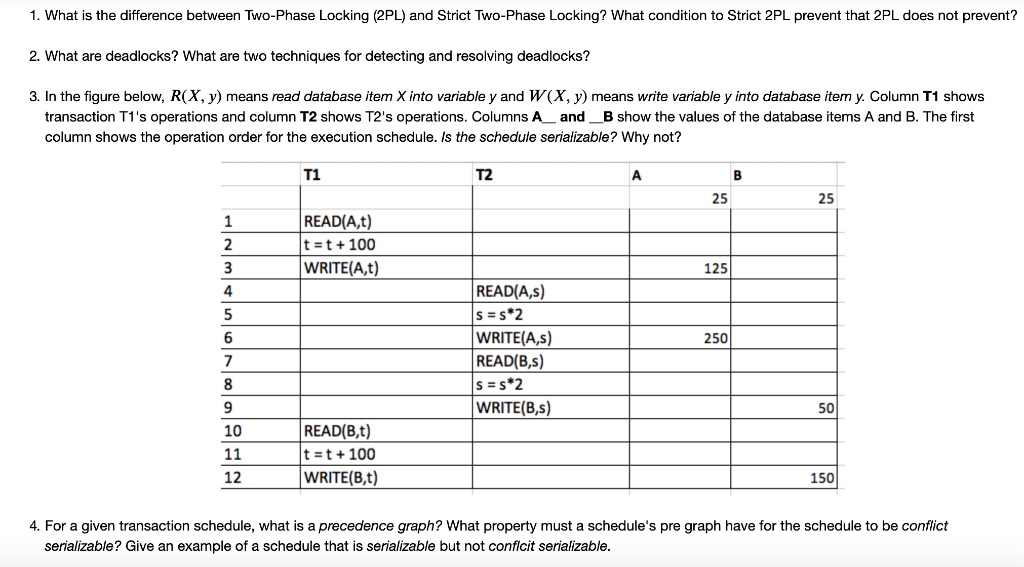1. What is the difference between Two-Phase Locking (2PL) and Strict Two-Phase Locking? What condition to Strict 2PL prevent that 2PL does not prevent? 2. What are deadlocks? What are two techniques for detecting and resolving deadlocks? 3. In the figure below, R(X, y) means read database item X into variable y and W(X, y) means write variable y into database item y. Column T1 shows transaction T1's operations and column T2 shows T2's operations. Columns Aand_B show the values...

• ### EXERCISE 2 [2.5/10] Given the following vector subspaces: W, Ξ {(x, y, z) E R3 / 0) x + y a) [1.0/10] Calculate base...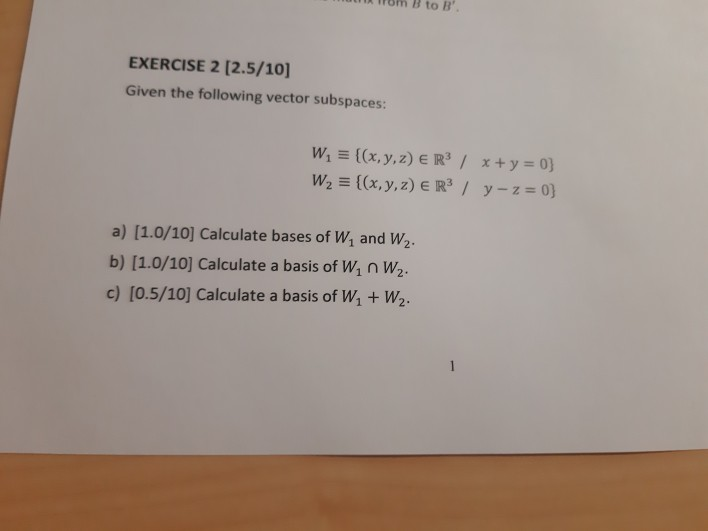EXERCISE 2 [2.5/10] Given the following vector subspaces: W, Ξ {(x, y, z) E R3 / 0) x + y a) [1.0/10] Calculate bases of Wi and W2. b) [1.0/10] Calculate a basis of W1 n W2 c) [0.5/10] Calculate a basis of W1 + W2 EXERCISE 2 [2.5/10] Given the following vector subspaces: W, Ξ {(x, y, z) E R3 / 0) x + y a) [1.0/10] Calculate bases of Wi and W2. b) [1.0/10] Calculate a basis of...

• ### 2. Consider the following transformations of R2 Tİ (z, y) (-r, y), T3(x, y) (z, _y), T,(zw) (y, x). Show that, for any j 1,2,3, a subset A C R2 is a Jordan region if and only if T,(A) is a Jordan...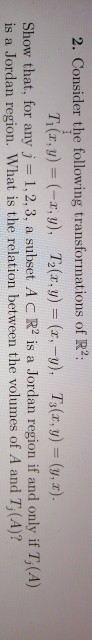2. Consider the following transformations of R2 Tİ (z, y) (-r, y), T3(x, y) (z, _y), T,(zw) (y, x). Show that, for any j 1,2,3, a subset A C R2 is a Jordan region if and only if T,(A) is a Jordan region. What is the relation between the volumes of A and T, (A)? 2. Consider the following transformations of R2 Tİ (z, y) (-r, y), T3(x, y) (z, _y), T,(zw) (y, x). Show that, for any j 1,2,3,...

• ### 2. a)Write the ARM ALP conditional code snippet for the following statements written in C-language. Assume R1 to Rn as06 variables Let R1, R2, R3 contain the starting addresses of arrays X, Y and Z r...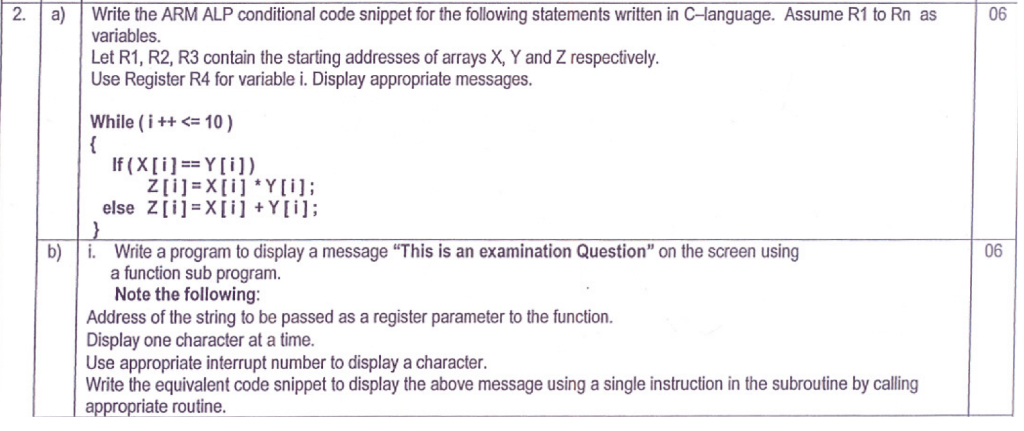2. a)Write the ARM ALP conditional code snippet for the following statements written in C-language. Assume R1 to Rn as06 variables Let R1, R2, R3 contain the starting addresses of arrays X, Y and Z respectively Use Register R4 for variable i. Display appropriate messages. While (i+10) else Z[i] XiYi; b)i Write a program to display a message "This is an examination Question" on the screen using 06 a function sub program Note the following Address of the string to...

Need Online Homework Help?